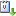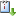Usted está aquí: The partition problem: an invitation to abstract convexity

# The partition problem: an invitation to abstract convexity

Ponente: Pierre Duchet
Institución: CNRS Paris, Francia
Tipo de Evento: Investigación
Cuándo 31/01/2017 de 17:00 a 18:00 Unidad Multidisciplinaria de Docencia e Investigación (UMDI), Aula 2. UNAM Campus Juriquilla, QuerétarovCaliCal
1. Given an integer k>1, every large set of points in R^d can be partitioned into k subsets whose convex hulls have a common point. Observe for instance that, with 9 points in the plane, we always can form 3 triples such that the resulting triangles share a common point. Observe that already with 7 points in general position in the plane we have the following striking property: the convex polygons formed with any 5 of those 7 points have a non empty intersection.

Actually those "partition properties" are far from being typical of Euclidean spaces. They hold, under very weak and general assumptions, in any "geometric" space where a reasonable notion of convex set can be defined. The partition problem consist in giving a purely combinatorial proof of a partition property into k parts ("Tverberg type" property) assuming only a partition property into 2 parts ("Radon type" property).

In this lecture, we present the general framework of abstract convexity spaces, focusing on the most important "dimensional" parameters, of combinatorial flavor, that can be associated to them. Those parameters are inspired by famous combinatorial theorems concerning convex sets in R^d: Kirchberger, Carathéodory, Helly, Radon, Krasnocelski, Tverberg... Theorems that marked the history of convexity in linear spaces and have received numerous variants, generalizations, leading to important applications to various domains of pure or applied mathematics (combinatorial geometry, functional analysis, game theory, discrete optimization and operation research).

We will discuss the central role of Radon theorem (the case k=2 of the partition property) in general abstract convexity spaces, with Helly type theorem and Tverberg type theorem as consequences. We examine the particular case of graphs, endowed with the natural convexity defined by shortest path containment. We prove that, finite graphs constitute, in some sense, a universal model for the partition problem: for any convexity space (E,C) with Helly number h and partition numbers p_k, with p_2>3, one may construct a graph G for which the shortest path convexity has Helly number h, Radon number p_2 and whose k-th partition number is at least p_k.